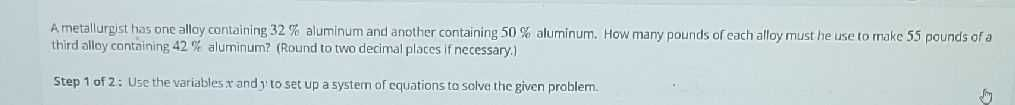### ¿Todavía tienes preguntas de matemáticas?

Pregunte a nuestros tutores expertos
Algebra
PreguntaA metallurgist thas one alloy containing $$32 \%$$ aluminum and another containing $$50 \%$$ aluminum. How many pounds of each alloy must he use to make $$55$$ pounds of a third alloy containing $$42 \%$$ aluminum? (Round to two decimal places if necessary.)

step $$1$$ of $$2$$ : Use the variables $$x$$ and y to set up a system of equations to solve the given problem.